Courses

# Fluid Mechanics - 1

## 15 Questions MCQ Test GATE Mechanical (ME) 2022 Mock Test Series | Fluid Mechanics - 1

Description
This mock test of Fluid Mechanics - 1 for GATE helps you for every GATE entrance exam. This contains 15 Multiple Choice Questions for GATE Fluid Mechanics - 1 (mcq) to study with solutions a complete question bank. The solved questions answers in this Fluid Mechanics - 1 quiz give you a good mix of easy questions and tough questions. GATE students definitely take this Fluid Mechanics - 1 exercise for a better result in the exam. You can find other Fluid Mechanics - 1 extra questions, long questions & short questions for GATE on EduRev as well by searching above.
QUESTION: 1

Solution:
QUESTION: 2

Solution:
QUESTION: 3

###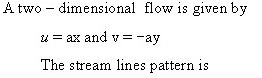Solution:
QUESTION: 4

The Bernoulli s equation is written with usual rotation as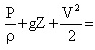constant

In this equation each of the terms represents.

Solution:
QUESTION: 5

The highest point of syphon is called as

Solution:
QUESTION: 6

If a rectangular plate 4m x 3m is completely submerged under a fluid of relative density 0.8 as
shown in the given figure, then the hydraulic thrust on the plate would be, (Take g= 10m/s2 )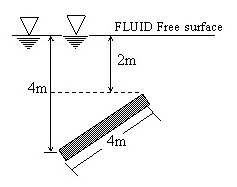Solution:
QUESTION: 7

Figure shows a vertical contraction pipe carries water. The water diameter D2 for the condition of P1 = P2 ,in meters (Neglect losses ) is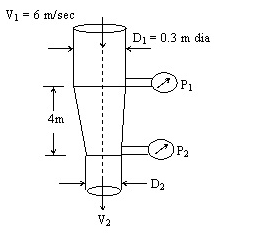Solution:
QUESTION: 8

The fluid entering a control volume has specific volume 0.833 m3/kg and a velocity of 5 m/sec, the area of the intake being 20 cm2 . If the fluid leaves the control volume through a 25 mm diameter pipe with a velocity of4 m/sec, the mass flow rate (in kg/sec)
and fluid mass density (in kg/m3 ) respectively are:

Solution:
QUESTION: 9

A ram - jet rocket engine consumes 20 kg of air/sec and 0.6 kg of fuel/sec. The exit velocity of the gases is 520 m/sec relative to the engine and the rocket velocity is 200 m/sec absolute. What is the nearly momentum power developed?

Solution:
*Answer can only contain numeric values
QUESTION: 10

A pitot - static tube is used to measure air velocity. If a manometer connected to the instrument indicates a difference in pressure head between the tappings of 4 mm of water, calculate the air velocity assuming the coefficient of the pitot tube to be unity. Take density of air = 1.2 kg/mand g = 9.8 m/sec2 .

The velocity of air is  ____  m/sec . ?

Solution:
*Answer can only contain numeric values
QUESTION: 11

Water is pumped through a pipe line to a height of 10 m at the rate of 6000 l.p.m, Friction and minor losses are 5m, then the power of pump required is ____ (in KW)
(Take g=10m/s2)

Solution:
QUESTION: 12

Match list -I with list -II and choose correct code.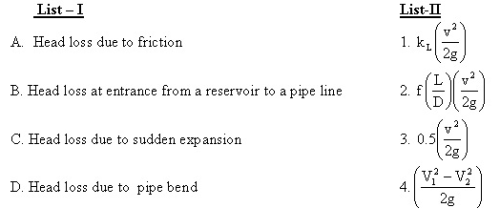Solution:
QUESTION: 13

In an automobile traveling at ‘V’ kmph the power expended in over coming resistance is T. If the automobile travels at ‘3 V’ kmph the power expended in over coming wind resistance is,

Solution:
QUESTION: 14

Water is flowing through a horizontal pipe of constant diameter and the flow is laminar. If the diameter of the pipe is increased by 50% keeping the volume flowrate constant, them the pressure drop in the pipe due to friction will decrease by

Solution:
QUESTION: 15

The head loss in 100 m length of a 0.1 m dia. pipe (f = 0.02) carrying water is 10 m. The boundary shear stress in kPa

Solution: